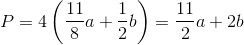## Example Questions

### Example Question #11 : Rhombuses

If a side length of a rhombus is half, what is the perimeter of the rhombus?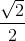Explanation:

Write the formula to find the perimeter of a rhombus.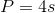Substitute the side length and simplify.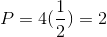### Example Question #2 : How To Find The Perimeter Of A Rhombus

Find the perimeter of a rhombus if the side length is.Explanation:

Write the formula to find the perimeter of a rhombus.Substitute the side length and solve.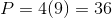### Example Question #12 : Rhombuses

If the side length of a rhombus is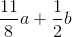, what is the perimeter?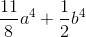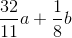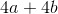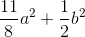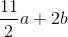Explanation:

Write the formula for the perimeter of a rhombus.Substitute the side length and solve for the perimeter.